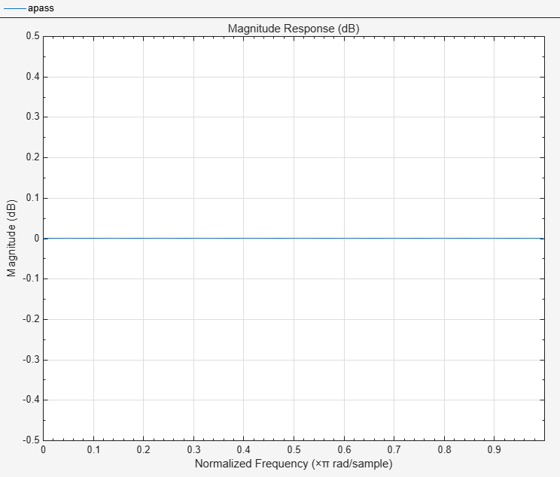# isallpass

Verify that discrete-time filter System object is allpass

## Syntax

``flag = isallpass(sysobj)``
``flag = isallpass(sysobj,tol)``
``flag = isallpass(___,'Arithmetic',arithType)``

## Description

example

````flag = isallpass(sysobj)` returns `true` if the filter System object™ is allpass.```
````flag = isallpass(sysobj,tol)` uses the tolerance `tol` to determine when two numbers are close enough to be considered equal.```
````flag = isallpass(___,'Arithmetic',arithType)` analyzes the filter System object based on the arithmetic specified in `arithType`, using either of the previous syntaxes.For more input options, see `isallpass` in Signal Processing Toolbox™.```

## Examples

collapse all

Create an allpass filter using the `dsp.AllpassFilter` object.

`apass = dsp.AllpassFilter`
```apass = dsp.AllpassFilter with properties: Structure: 'Minimum multiplier' AllpassCoefficients: [-0.7071 0.5000] TrailingFirstOrderSection: false ```

Verify that the filter is an allpass filter by visualizing the magnitude response in `fvtool`.

`fvtool(apass)`Now verify that the filter is an allpass filter using the `isallpass` function.

`isallpass(apass)`
```ans = logical 1 ```

## Input Arguments

collapse all

Tolerance value to determine when two numbers are close enough to be considered equal, specified as a positive scalar. If not specified, `tol`, defaults to `eps^(2/3)`. Specifying a tolerance may be most helpful in fixed-point allpass filters.

Arithmetic used in the filter analysis, specified as `'double'`, `'single'`, or `'Fixed'`. When the arithmetic input is not specified and the filter System object is unlocked, the analysis tool assumes a double-precision filter. When the arithmetic input is not specified and the System object is locked, the function performs the analysis based on the data type of the locked input.

The `'Fixed'` value applies to filter System objects with fixed-point properties only.

When the `'Arithmetic'` input argument is specified as `'Fixed'` and the filter object has the data type of the coefficients set to `'Same word length as input'`, the arithmetic analysis depends on whether the System object is unlocked or locked.

• unlocked –– The analysis object function cannot determine the coefficients data type. The function assumes that the coefficients data type is signed, has a 16-bit word length, and is auto scaled. The function performs fixed-point analysis based on this assumption.

• locked –– When the input data type is `'double'` or `'single'`, the analysis object function cannot determine the coefficients data type. The function assumes that the data type of the coefficients is signed, has a 16-bit word length, and is auto scaled. The function performs fixed-point analysis based on this assumption.

To check if the System object is locked or unlocked, use the `isLocked` function.

When the arithmetic input is specified as `'Fixed'` and the filter object has the data type of the coefficients set to a custom numeric type, the object function performs fixed-point analysis based on the custom numeric data type.

## Output Arguments

collapse all

Flag to determine if the filter is allpass, returned as a logical:

• `1` –– Filter is allpass.

• `0` –– Filter is not allpass.

Data Types: `logical`